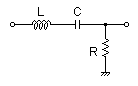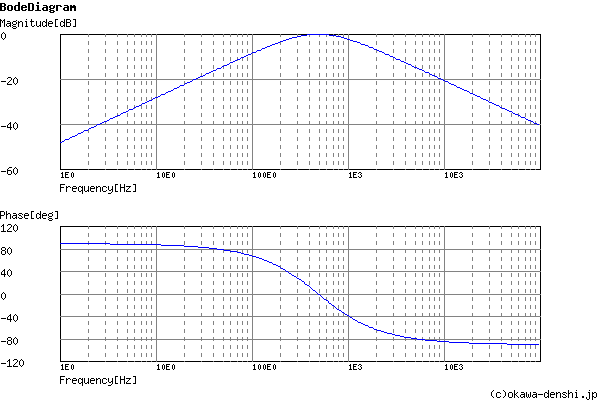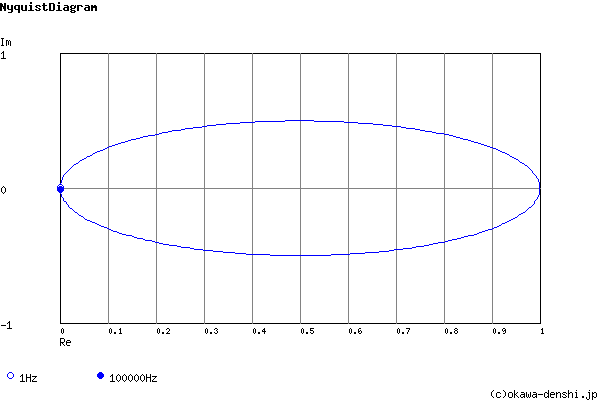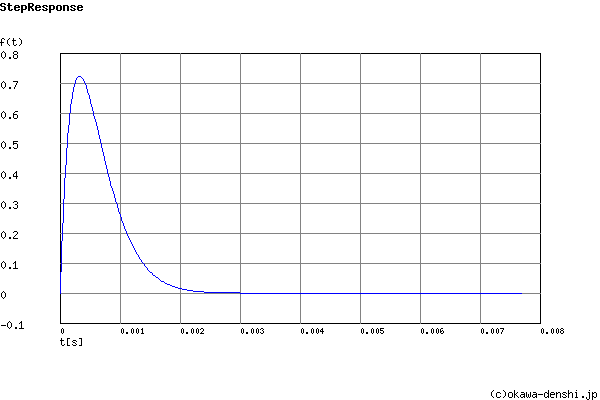# (Sample)RLC Band-pass Filter Design Tool - Result -

Calculated the transfer function for the RLC Band-pass filter, displayed on graphs, showing Bode diagram, Nyquist diagram, Impulse response and Step response.

## RLC Filter

 Vin(s)→→Vout(s)
(Sample)Transfer Function:
 G(s)= 5909.09090909s s2+5909.09090909s+9671179.88395

R = 13Ω
C = 47uF
L = 0.0022H

#### Center passes frequency

f0 = 494.948328884[Hz]

#### Quality factor

Q = 0.526282657637

#### Damping ratio

ζ = 0.95005980673

#### Pole(s)

p = -470.230513681 +154.457477218i[Hz]
|p|= 494.948328884[Hz]
p = -470.230513681-154.457477218i[Hz]
|p|= 494.948328884[Hz]

z = 0[Hz]
|z|= 0[Hz]

#### Phase margin

pm= NAN[deg] (f =0[Hz])

#### Oscillation frequency

f = 154.457477218[Hz]

#### Overshoot (in absolute value)

The 1st peak  gpk = 0.72 (t =0.00031[sec])
The 2nd peak  gpk = -5.1E-05 (t =0.0036[sec])
The 3rd peak  gpk = 3.6E-09 (t =0.0068[sec])

g(∞) = 0

### Q factor | Damping ratio ζ

Quality factor Q =
Damping ratio  ζ =
 fc= Hz L = H C = F
Give two values from three parameters of f0 ,L, C.

Select Capacitor Sequence:
Select Resistor Sequence:
Select Inductance Sequence:

### Frequency analysis

Bode diagram
Phase  Group delay
Nyquist diagram
Pole, zero
Phase margin
Oscillation analysis
Analysis on frequency range:
f1=∼f2=[Hz] (optional)

### Transient analysis

Step response
Impulse response
Overshoot
Final value of the step response
Analysis on time range:
0∼[sec] (optional)

# Frequency analysis# Transient analysis